# Wall-Sun-Sun primes and Fibonacci divisibility

 Importance: Medium ✭✭
 Author(s):
 Subject: Number Theory » Computational Number Theory
 Keywords: Fibonacci prime
 Posted by: adudzik on: June 14th, 2008
Conjecture   For any prime, there exists a Fibonacci number divisible byexactly once.

Equivalently:

Conjecture   For any prime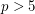,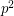does not dividewhere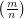is the Legendre symbol.

Letbe an odd prime, and let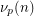denote the-adic valuation of. Letbe the smallest Fibonacci number that is divisible by(which must exist by a simple counting argument). A well-known result says thatunless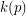divides, and. This conjecture asserts that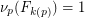for all. This has been verified up to at least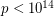. [EJ]

This conjecture is equivalent to non-existence of Wall-Sun-Sun primes.

## Bibliography

[EJ] Andreas-Stephan Elsenhansand and Jörg Jahnel, The Fibonacci sequence modulo p^2

[R] Marc Renault, Properties of the Fibonacci Sequence Under Various Moduli

*[W] D. D. Wall, Fibonacci Series Modulo m, American Mathematical Monthly, 67 (1960), pp. 525-532.

* indicates original appearance(s) of problem.